Calculus Volume 2

# Chapter 4

### Checkpoint

4.2

$5 5$

4.3

$y = 2 x 2 + 3 x + 2 y = 2 x 2 + 3 x + 2$

4.5

$y = 1 3 x 3 − 2 x 2 + 3 x − 6 e x + 14 y = 1 3 x 3 − 2 x 2 + 3 x − 6 e x + 14$

4.6

$v ( t ) = −9.8 t v ( t ) = −9.8 t$

4.7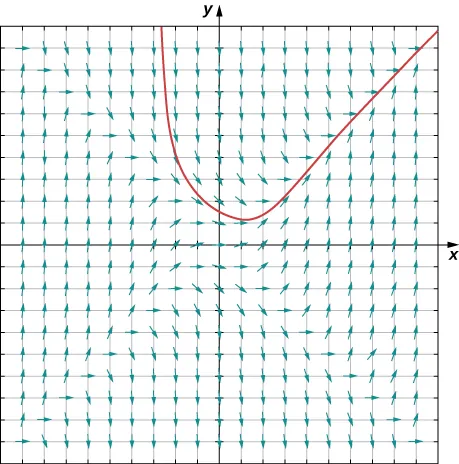4.8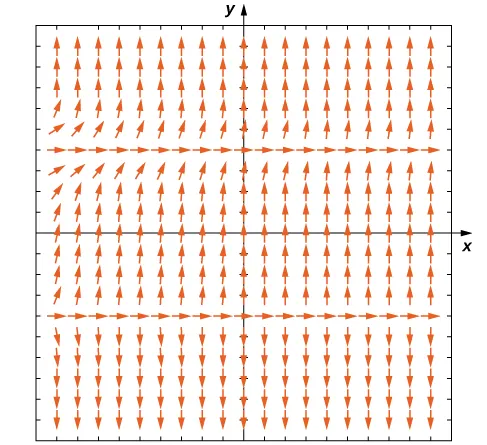The equilibrium solutions are $y=−2y=−2$ and $y=2.y=2.$ For this equation, $y=−2y=−2$ is an unstable equilibrium solution, and $y=2y=2$ is a semi-stable equilibrium solution.

4.9
$nn$ $xnxn$ $yn=yn−1+hf(xn−1,yn−1)yn=yn−1+hf(xn−1,yn−1)$
$00$ $11$ $−2−2$
$11$ $1.11.1$ $y1=y0+hf(x0,y0)=−1.5y1=y0+hf(x0,y0)=−1.5$
$22$ $1.21.2$ $y2=y1+hf(x1,y1)=−1.1419y2=y1+hf(x1,y1)=−1.1419$
$33$ $1.31.3$ $y3=y2+hf(x2,y2)=−0.8387y3=y2+hf(x2,y2)=−0.8387$
$44$ $1.41.4$ $y4=y3+hf(x3,y3)=−0.5487y4=y3+hf(x3,y3)=−0.5487$
$55$ $1.51.5$ $y5=y4+hf(x4,y4)=−0.2442y5=y4+hf(x4,y4)=−0.2442$
$66$ $1.61.6$ $y6=y5+hf(x5,y5)=0.0993y6=y5+hf(x5,y5)=0.0993$
$77$ $1.71.7$ $y7=y6+hf(x6,y6)=0.5099y7=y6+hf(x6,y6)=0.5099$
$88$ $1.81.8$ $y8=y7+hf(x7,y7)=1.0272y8=y7+hf(x7,y7)=1.0272$
$99$ $1.91.9$ $y9=y8+hf(x8,y8)=1.7159y9=y8+hf(x8,y8)=1.7159$
$1010$ $22$ $y10=y9+hf(x9,y9)=2.6962y10=y9+hf(x9,y9)=2.6962$
4.10

$y = 2 + C e x 2 + 3 x y = 2 + C e x 2 + 3 x$

4.11

$y = 4 + 14 e x 2 + x 1 − 7 e x 2 + x y = 4 + 14 e x 2 + x 1 − 7 e x 2 + x$

4.12

Initial value problem:

$d u d t = 2.4 − 2 u 25 , u ( 0 ) = 3 d u d t = 2.4 − 2 u 25 , u ( 0 ) = 3$

$Solution: u ( t ) = 30 − 27 e − t / 50 Solution: u ( t ) = 30 − 27 e − t / 50$

4.13
1. Initial-value problem
$dTdt=k(T−70),T(0)=450dTdt=k(T−70),T(0)=450$
2. $T(t)=70+380ektT(t)=70+380ekt$
3. Approximately $114114$ minutes.
4.14
1. $dPdt=0.04(1−P750),P(0)=200dPdt=0.04(1−P750),P(0)=200$

2.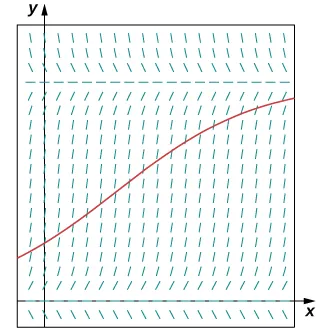3. $P(t)=3000e.04t11+4e.04tP(t)=3000e.04t11+4e.04t$

4. After $1212$ months, the population will be $P(12)≈278P(12)≈278$ rabbits.

4.15

$y′+15x+3y=10x−20x+3;p(x)=15x+3y′+15x+3y=10x−20x+3;p(x)=15x+3$ and $q(x)=10x−20x+3q(x)=10x−20x+3$

4.16

$y = x 3 + x 2 + C x − 2 y = x 3 + x 2 + C x − 2$

4.17

$y = - 2 x - 5 2 + 1 2 e 2 x y = - 2 x - 5 2 + 1 2 e 2 x$

4.18
1. $dvdt=−v−9.8v(0)=0dvdt=−v−9.8v(0)=0$
2. $v(t)=9.8(e−t−1)v(t)=9.8(e−t−1)$
3. $limt→∞v(t)=limt→∞(9.8(e−t−1))=−9.8m/s≈−21.922mphlimt→∞v(t)=limt→∞(9.8(e−t−1))=−9.8m/s≈−21.922mph$
4.19

Initial-value problem:

$8 q ′ + 1 0.02 q = 20 sin 5 t , q ( 0 ) = 4 8 q ′ + 1 0.02 q = 20 sin 5 t , q ( 0 ) = 4$

$q ( t ) = 10 sin 5 t − 8 cos 5 t + 172 e −6.25 t 41 q ( t ) = 10 sin 5 t − 8 cos 5 t + 172 e −6.25 t 41$

### Section 4.1 Exercises

1.

$1 1$

3.

$3 3$

5.

$1 1$

7.

$1 1$

19.

$y = 4 + 3 x 4 4 y = 4 + 3 x 4 4$

21.

$y = 1 2 e x 2 y = 1 2 e x 2$

23.

$y = 2 e − 1 / x y = 2 e − 1 / x$

25.

$u = sin −1 ( e −1 + t ) u = sin −1 ( e −1 + t )$

27.

$y = − x + 1 1 − x − 1 y = − x + 1 1 − x − 1$

29.

$y = C − x + x ln x − ln ( cos x ) y = C − x + x ln x − ln ( cos x )$

31.

$y = C + 4 x ln ( 4 ) y = C + 4 x ln ( 4 )$

33.

$y = 2 3 t 2 + 16 ( t 2 + 16 ) + C y = 2 3 t 2 + 16 ( t 2 + 16 ) + C$

35.

$x = 2 15 4 + t ( 3 t 2 + 4 t − 32 ) + C x = 2 15 4 + t ( 3 t 2 + 4 t − 32 ) + C$

37.

$y = C x y = C x$

39.

$y = 1 − t 2 2 , y = − t 2 2 − 1 y = 1 − t 2 2 , y = − t 2 2 − 1$

41.

$y = e − t , y = − e − t y = e − t , y = − e − t$

43.

$y = 2 ( t 2 + 5 ) , t = 3 5 y = 2 ( t 2 + 5 ) , t = 3 5$

45.

$y = 10 e −2 t , t = − 1 2 ln ( 1 10 ) y = 10 e −2 t , t = − 1 2 ln ( 1 10 )$

47.

$y=14(41−e−4t),y=14(41−e−4t),$ never

49.

Solution changes from increasing to decreasing at $y(0)=0y(0)=0$

51.

Solution changes from increasing to decreasing at $y(0)=0y(0)=0$

53.

$v ( t ) = −32 t + a v ( t ) = −32 t + a$

55.

$00$ ft/s

57.

$52.35452.354$ meters

59.

$x=50t−15π2cos(πt)+3π2,2x=50t−15π2cos(πt)+3π2,2$ hours $11$ minute

61.

$y = 4 e 3 t y = 4 e 3 t$

63.

$y = 3 − 2 t + t 2 y = 3 − 2 t + t 2$

65.

$y=1k(ekt−1)y=1k(ekt−1)$ and $y=xy=x$

### Section 4.2 Exercises

67.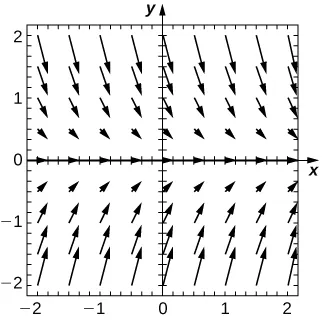69.

$y=0y=0$ is a stable equilibrium

71.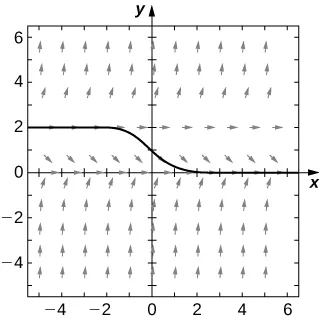73.

$y=0y=0$ is a stable equilibrium and $y=2y=2$ is unstable

75.

General solution is $y=et+Cy=et+C$.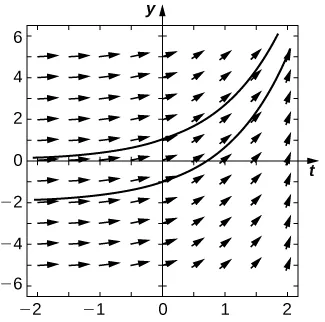77.

General solution is $y=et(t-1)+Cy=et(t-1)+C$.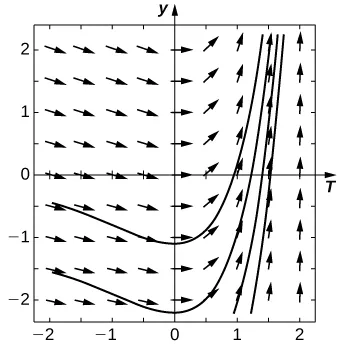79.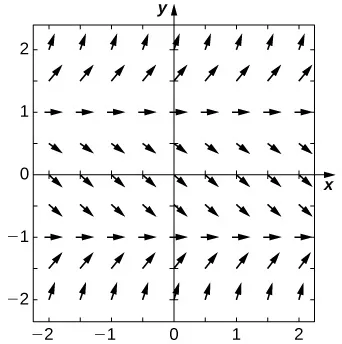81.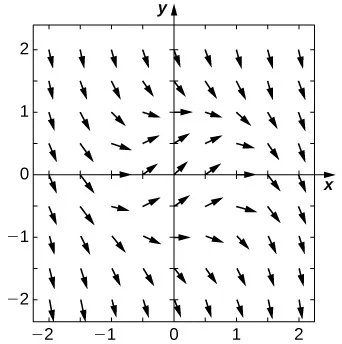83.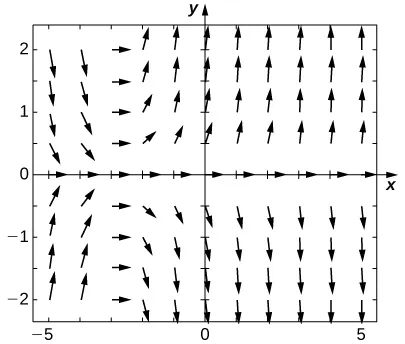85.

E

87.

A

89.

B

91.

A

93.

C

95.

$2.24,2.24,$ exact: $33$

97.

$7.739364,7.739364,$ exact: $5(e−1)5(e−1)$

99.

$−0.2535−0.2535$ exact: $00$

101.

$1.345,1.345,$ exact: $1ln(2)1ln(2)$

103.

$−4,−4,$ exact: $−1/2−1/2$

105.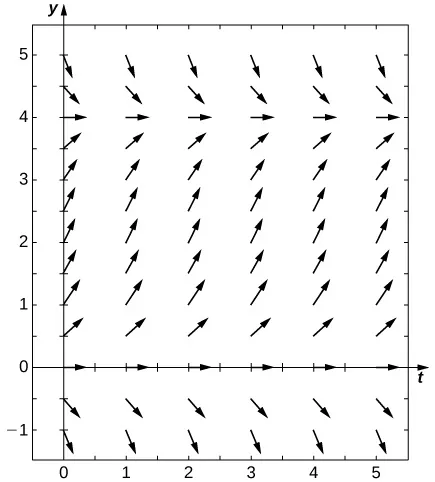107.

$y ′ = 2 e t 2 / 2 y ′ = 2 e t 2 / 2$

109.

$2 2$

111.

$3.2756 3.2756$

113.

$2e2e$

Step Size Relative Error
$h=0.1h=0.1$ $0.39350.3935$
$h=0.01h=0.01$ $0.061630.06163$
$h=0.001h=0.001$ $0.0066120.006612$
$h=0.0001h=0.0001$ $0.00066610.0006661$
115.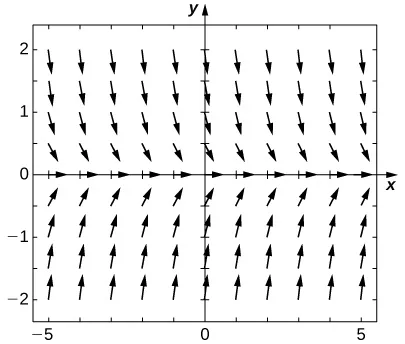117.

$4.0741 e −10 4.0741 e −10$

### Section 4.3 Exercises

119.

$y = e t − 1 y = e t − 1$

121.

$y = 1 + C e − t y = 1 + C e − t$

123.

$y = C x e −1 / x y = C x e −1 / x$

125.

$y = 1 C − x 2 y = 1 C − x 2$

127.

$y = − 2 C + ln x y = − 2 C + ln x$

129.

$y = C e x ( x + 1 ) + 1 y = C e x ( x + 1 ) + 1$

131.

$y = sin ( ln t + C ) y = sin ( ln t + C )$

133.

$y = − ln ( e − x ) y = − ln ( e − x )$

135.

$y = 1 2 − e x 2 y = 1 2 − e x 2$

137.

$y = tanh −1 ( x 2 2 ) y = tanh −1 ( x 2 2 )$

139.

$x = sin ( 1 - t + t ln t ) x = sin ( 1 - t + t ln t )$

141.

$y = ln ( ln ( 5 ) ) − ln ( 2 − 5 x ) y = ln ( ln ( 5 ) ) − ln ( 2 − 5 x )$

143.

$y=Ce−2x+12y=Ce−2x+12$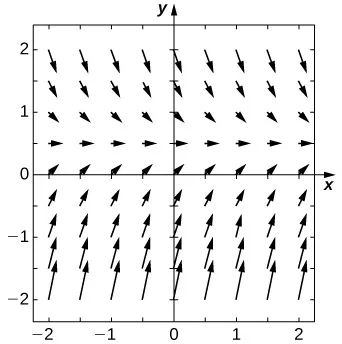145.

$y=12C−exy=12C−ex$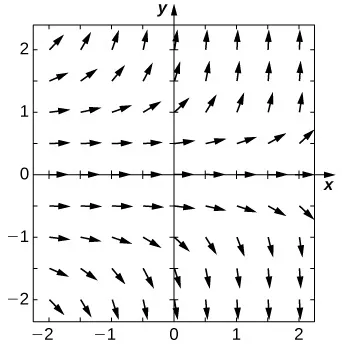147.

$y=Ce−xxxy=Ce−xxx$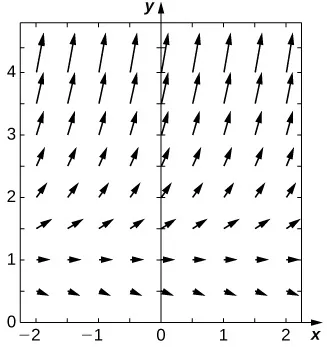149.

$y = r d ( 1 − e − d t ) y = r d ( 1 − e − d t )$

151.

$y ( t ) = 10 − 9 e − x / 50 y ( t ) = 10 − 9 e − x / 50$

153.

$134.3134.3$ kilograms

155.

$720720$ seconds

157.

$2424$ hours $5757$ minutes

159.

$T ( t ) = 20 + 50 e −0.125 t T ( t ) = 20 + 50 e −0.125 t$

161.

$T ( t ) = 20 + 38.5 e −0.125 t T ( t ) = 20 + 38.5 e −0.125 t$

163.

$y = ( c + b a ) e a x − b a y = ( c + b a ) e a x − b a$

165.

$y ( t ) = c L + ( I − c L ) e − r t / L y ( t ) = c L + ( I − c L ) e − r t / L$

167.

$y=40(1−e−0.1t),40y=40(1−e−0.1t),40$ g/cm2

### Section 4.4 Exercises

169.$P=0P=0$ semi-stable

171.

$P = 10 e 10 x e 10 x + 4 P = 10 e 10 x e 10 x + 4$

173.

$P ( t ) = 10000 e 0.02 t 150 + 50 e 0.02 t P ( t ) = 10000 e 0.02 t 150 + 50 e 0.02 t$

175.

$6969$ hours $55$ minutes

177.

$88$ years $1111$ months

179.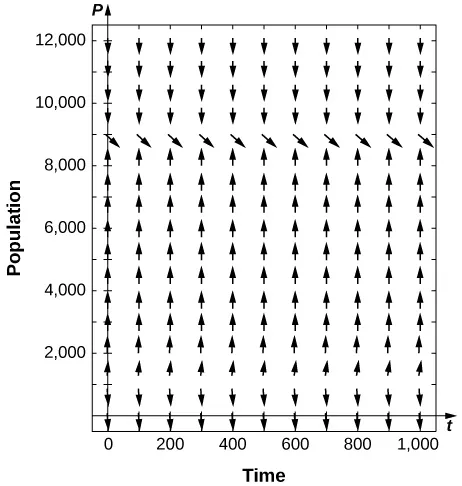181.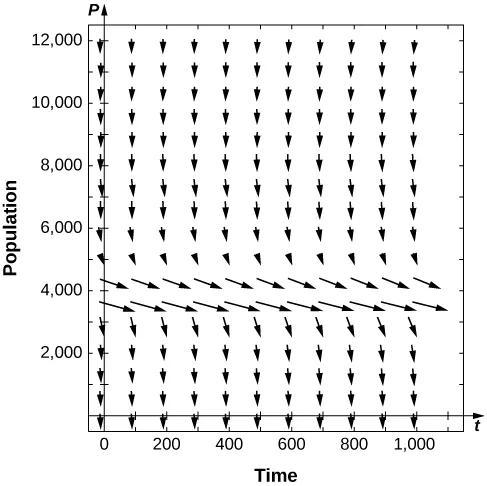$P1P1$ semi-stable

183.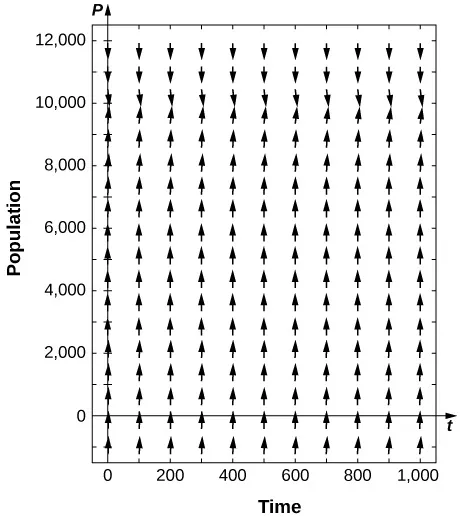$P2>0P2>0$ stable

185.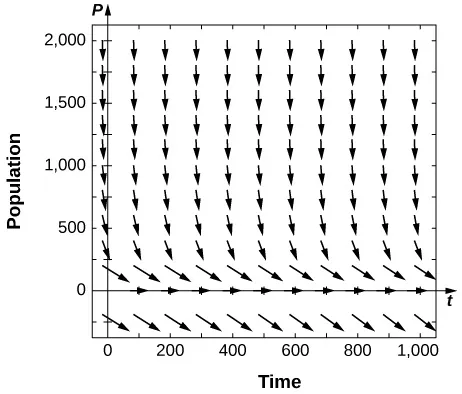$P1=0P1=0$ is semi-stable

187.

$y = −20 4 × 10 −6 − 0.002 e 0.01 t y = −20 4 × 10 −6 − 0.002 e 0.01 t$

189.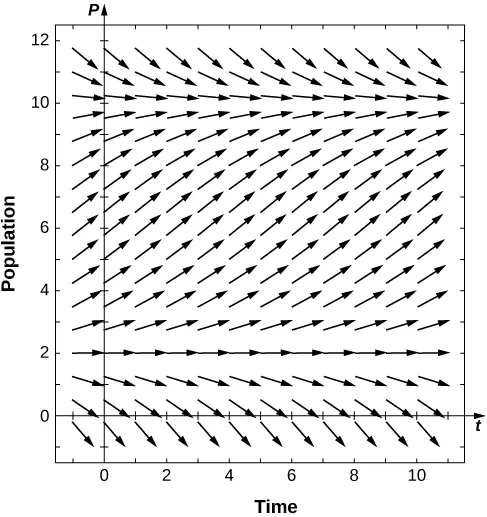191.

$P ( t ) = 850 + 500 e 0.009 t 85 + 5 e 0.009 t P ( t ) = 850 + 500 e 0.009 t 85 + 5 e 0.009 t$

193.

$1313$ years months

195.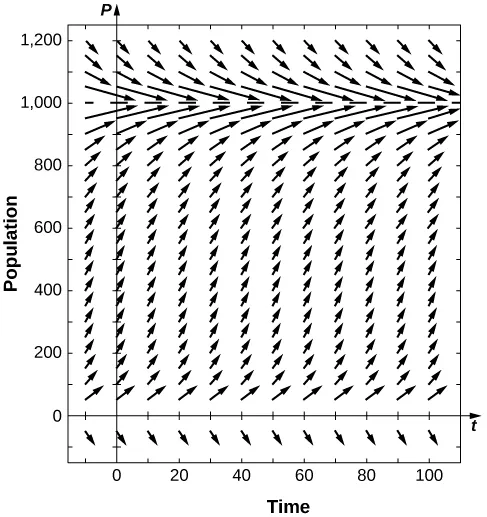197.

$31.46531.465$ days

199.

September $20082008$

201.

$K + T 2 K + T 2$

203.

$r = 0.0405 r = 0.0405$

205.

$α = 0.0081 α = 0.0081$

207.

Logistic: $361,361,$ Threshold: $436,436,$ Gompertz: $309.309.$

### Section 4.5 Exercises

209.

Yes

211.

Yes

213.

$y ′ − x 3 y = sin x y ′ − x 3 y = sin x$

215.

$y ′ + ( 3 x + 2 ) x y = − e x y ′ + ( 3 x + 2 ) x y = − e x$

217.

$d y d t − y x ( x + 1 ) = 0 d y d t − y x ( x + 1 ) = 0$

219.

$e x e x$

221.

$− ln ( cosh x ) − ln ( cosh x )$

223.

$y = C e 3 x − 2 3 y = C e 3 x − 2 3$

225.

$y = C x 3 + 6 x 2 y = C x 3 + 6 x 2$

227.

$y = C e x 2 / 2 − 3 y = C e x 2 / 2 − 3$

229.

$y = C tan ( x 2 ) − 2 x + 4 tan ( x 2 ) ln ( sin ( x 2 ) ) y = C tan ( x 2 ) − 2 x + 4 tan ( x 2 ) ln ( sin ( x 2 ) )$

231.

$y = C x 3 − x 2 y = C x 3 − x 2$

233.

$y = C ( x + 2 ) 2 + 1 2 y = C ( x + 2 ) 2 + 1 2$

235.

$y = C x + 2 sin ( 3 t ) y = C x + 2 sin ( 3 t )$

237.

$y = C ( x + 1 ) 3 − x 2 − 2 x − 1 y = C ( x + 1 ) 3 − x 2 − 2 x − 1$

239.

$y = C e sinh −1 x − 2 y = C e sinh −1 x − 2$

241.

$y = x + 4 e x − 1 y = x + 4 e x − 1$

243.

$y = − 3 x 2 ( x 2 − 1 ) y = − 3 x 2 ( x 2 − 1 )$

245.

$y = 1 − e tan −1 x y = 1 − e tan −1 x$

247.

$y = ( x + 2 ) ln ( x + 2 2 ) y = ( x + 2 ) ln ( x + 2 2 )$

249.

$y = 2 e 2 x − 2 x − 2 x − 1 y = 2 e 2 x − 2 x − 2 x − 1$

251.

$v ( t ) = g m k ( 1 − e − k t / m ) v ( t ) = g m k ( 1 − e − k t / m )$

253.

$40.45140.451$ seconds

255.

$g m k g m k$

257.

$y = C e x − a ( x + 1 ) y = C e x − a ( x + 1 )$

259.

$y = C e x 2 / 2 − a y = C e x 2 / 2 − a$

261.

$y = e k t − e t k − 1 y = e k t − e t k − 1$

### Review Exercises

263.

F

265.

T

267.

$y ( x ) = 2 x ln ( 2 ) + x cos −1 x − 1 − x 2 + C y ( x ) = 2 x ln ( 2 ) + x cos −1 x − 1 − x 2 + C$

269.

$y ( x ) = ln ( C − cos x ) y ( x ) = ln ( C − cos x )$

271.

$y ( x ) = e e C + x y ( x ) = e e C + x$

273.

$y ( x ) = 4 + 3 2 x 2 + 2 x − sin x y ( x ) = 4 + 3 2 x 2 + 2 x − sin x$

275.

$y ( x ) = − 2 1 + 3 ( x 2 + 2 sin x ) y ( x ) = − 2 1 + 3 ( x 2 + 2 sin x )$

277.

$y ( x ) = −2 x 2 − 2 x − 1 3 − 2 3 e 3 x y ( x ) = −2 x 2 − 2 x − 1 3 − 2 3 e 3 x$

279.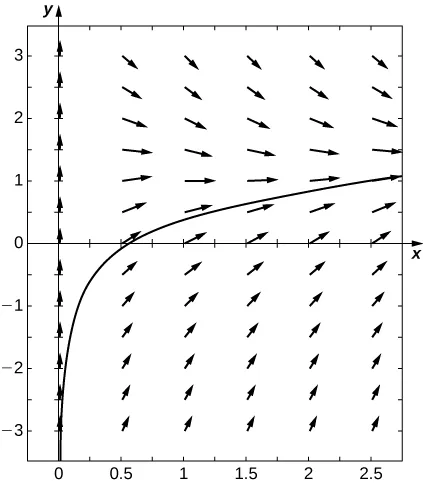$y(x)=Ce−x+lnxy(x)=Ce−x+lnx$

281.

Euler: $0.6939,0.6939,$ exact solution: $y(x)=3x−e−2x2+ln(3)y(x)=3x−e−2x2+ln(3)$

283.

$40494049$ second

285.

$x(t)=5000+2459−493t−2459e−5/3t,t=307.8x(t)=5000+2459−493t−2459e−5/3t,t=307.8$ seconds

287.

$T ( t ) = 200 ( 1 − e − t / 1000 ) T ( t ) = 200 ( 1 − e − t / 1000 )$

289.

$P ( t ) = 1600000 e 0.02 t 9840 + 160 e 0.02 t P ( t ) = 1600000 e 0.02 t 9840 + 160 e 0.02 t$

Order a print copy

As an Amazon Associate we earn from qualifying purchases.